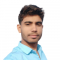## Found 3 Articles for AddersUpdated on 19-Apr-2023 15:42:38
Let us stat this article with a brief introduction of binary adders and rules of binary addition. In digital electronics, an adder or binary adder is a combinational digital circuit which performs the addition of two or more binary digits. The binary addition of two bits is performed by following these four rules − 0 + 0 = 0 0 + 1 = 1 ... Read More

## Adders and Subtractors in Digital ElectronicsUpdated on 26-Dec-2022 17:39:27
In digital electronics, adders and subtractors both are the combinational logic circuits (a combinational logic circuit is one whose output depends only on the present inputs, but not on the past outputs) that can add or subtract numbers, more specifically binary numbers. Adders and subtractors are the crucial parts of arithmetic logic circuits in processing devices like microprocessors or microcontrollers. In this article, we will discuss adders and subtractors in detail. What is an Adder? We have different types of digital devices like computers, calculators that can perform a variety of processing functions like addition, subtraction, multiplication, division, etc. The ... Read More